Search by Topic

Filter by: Content type:
Age range:
Challenge level:

There are 56 NRICH Mathematical resources connected to Graphs, you may find related items under Functions and Graphs.

Broad Topics > Functions and Graphs > Graphs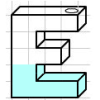Mathsjam Jars

Age 14 to 16 Challenge Level:

Imagine different shaped vessels being filled. Can you work out what the graphs of the water level should look like?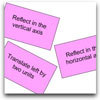Surprising Transformations

Age 14 to 16 Challenge Level:

I took the graph y=4x+7 and performed four transformations. Can you find the order in which I could have carried out the transformations?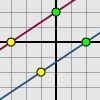Translating Lines

Age 11 to 14 Challenge Level:

Investigate what happens to the equation of different lines when you translate them. Try to predict what will happen. Explain your findings.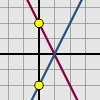Reflecting Lines

Age 11 to 14 Challenge Level:

Investigate what happens to the equations of different lines when you reflect them in one of the axes. Try to predict what will happen. Explain your findings.Immersion

Age 14 to 16 Challenge Level:

Various solids are lowered into a beaker of water. How does the water level rise in each case?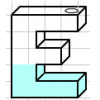Maths Filler

Age 11 to 14 Challenge Level:

Imagine different shaped vessels being filled. Can you work out what the graphs of the water level should look like?Equation Matcher

Age 16 to 18 Challenge Level:

Can you match these equations to these graphs?Real-life Equations

Age 16 to 18 Challenge Level:

Here are several equations from real life. Can you work out which measurements are possible from each equation?Diamond Collector

Age 11 to 14 Challenge Level:

Collect as many diamonds as you can by drawing three straight lines.Matchless

Age 14 to 16 Challenge Level:

There is a particular value of x, and a value of y to go with it, which make all five expressions equal in value, can you find that x, y pair ?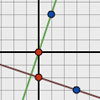Perpendicular Lines

Age 14 to 16 Challenge Level:

Position the lines so that they are perpendicular to each other. What can you say about the equations of perpendicular lines?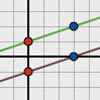Parallel Lines

Age 11 to 14 Challenge Level:

How does the position of the line affect the equation of the line? What can you say about the equations of parallel lines?Spaces for Exploration

Age 11 to 14

Alf Coles writes about how he tries to create 'spaces for exploration' for the students in his classrooms.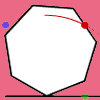Up and Across

Age 11 to 14 Challenge Level:

Experiment with the interactivity of "rolling" regular polygons, and explore how the different positions of the red dot affects its vertical and horizontal movement at each stage.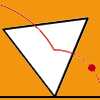Speeding Up, Slowing Down

Age 11 to 14 Challenge Level:

Experiment with the interactivity of "rolling" regular polygons, and explore how the different positions of the red dot affects its speed at each stage.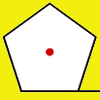How Far Does it Move?

Age 11 to 14 Challenge Level:

Experiment with the interactivity of "rolling" regular polygons, and explore how the different positions of the red dot affects the distance it travels at each stage.Exponential Trend

Age 16 to 18 Challenge Level:

Find all the turning points of y=x^{1/x} for x>0 and decide whether each is a maximum or minimum. Give a sketch of the graph.Fence It

Age 11 to 14 Challenge Level:

If you have only 40 metres of fencing available, what is the maximum area of land you can fence off?Walk and Ride

Age 7 to 14 Challenge Level:

How far have these students walked by the time the teacher's car reaches them after their bus broke down?Exploring Cubic Functions

Age 14 to 18 Challenge Level:

Quadratic graphs are very familiar, but what patterns can you explore with cubics?Parabolas Again

Age 14 to 18 Challenge Level:

Here is a pattern composed of the graphs of 14 parabolas. Can you find their equations?Parabella

Age 16 to 18 Challenge Level:

This is a beautiful result involving a parabola and parallels.Parabolic Patterns

Age 14 to 18 Challenge Level:

The illustration shows the graphs of fifteen functions. Two of them have equations y=x^2 and y=-(x-4)^2. Find the equations of all the other graphs.How Many Solutions?

Age 16 to 18 Challenge Level:

Find all the solutions to the this equation.Interpolating Polynomials

Age 16 to 18 Challenge Level:

Given a set of points (x,y) with distinct x values, find a polynomial that goes through all of them, then prove some results about the existence and uniqueness of these polynomials.What's That Graph?

Age 14 to 16 Challenge Level:

Can you work out which processes are represented by the graphs?Electric Kettle

Age 14 to 16 Challenge Level:

Explore the relationship between resistance and temperatureWhich Is Bigger?

Age 14 to 16 Challenge Level:

Which is bigger, n+10 or 2n+3? Can you find a good method of answering similar questions?Which Is Cheaper?

Age 14 to 16 Challenge Level:

When I park my car in Mathstown, there are two car parks to choose from. Can you help me to decide which one to use?Guess the Function

Age 16 to 18 Challenge Level:

This task depends on learners sharing reasoning, listening to opinions, reflecting and pulling ideas together.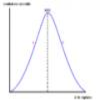Graphic Biology

Age 16 to 18 Challenge Level:

Several graphs of the sort occurring commonly in biology are given. How many processes can you map to each graph?Four on the Road

Age 14 to 16 Challenge Level:

Four vehicles travel along a road one afternoon. Can you make sense of the graphs showing their motion?Whose Line Graph Is it Anyway?

Age 16 to 18 Challenge Level:

Which line graph, equations and physical processes go together?Curve Match

Age 16 to 18 Challenge Level:

Which curve is which, and how would you plan a route to pass between them?Curve Fitter

Age 16 to 18 Challenge Level:

Can you fit a cubic equation to this graph?Maths Filler 2

Age 14 to 16 Challenge Level:

Can you draw the height-time chart as this complicated vessel fills with water?Bio Graphs

Age 14 to 16 Challenge Level:

What biological growth processes can you fit to these graphs?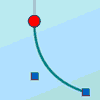Graphical Interpretation

Age 14 to 16 Challenge Level:

This set of resources for teachers offers interactive environments to support work on graphical interpretation at Key Stage 4.Motion Sensor

Age 14 to 16 Challenge Level:

Looking at the graph - when was the person moving fastest? Slowest?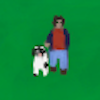Take Your Dog for a Walk

Age 7 to 11 Challenge Level:

Use the interactivity to move Mr Pearson and his dog. Can you move him so that the graph shows a curve?You Tell the Story

Age 7 to 11 Challenge Level:

Can you create a story that would describe the movement of the man shown on these graphs? Use the interactivity to try out our ideas.Golden Construction

Age 16 to 18 Challenge Level:

Draw a square and an arc of a circle and construct the Golden rectangle. Find the value of the Golden Ratio.Bus Stop

Age 14 to 16 Challenge Level:

Two buses leave at the same time from two towns Shipton and Veston on the same long road, travelling towards each other. At each mile along the road are milestones. The buses' speeds are constant. . . .Gosh Cosh

Age 16 to 18 Challenge Level:

Explore the hyperbolic functions sinh and cosh using what you know about the exponential function.Climbing

Age 16 to 18 Challenge Level:

Sketch the graphs of y = sin x and y = tan x and some straight lines. Prove some inequalities.Small Steps

Age 16 to 18 Challenge Level:

Two problems about infinite processes where smaller and smaller steps are taken and you have to discover what happens in the limit.Lap Times

Age 14 to 16 Challenge Level:

Can you find the lap times of the two cyclists travelling at constant speeds?Ellipses

Age 14 to 18 Challenge Level:

Here is a pattern for you to experiment with using graph drawing software. Find the equations of the graphs in the pattern.More Parabolic Patterns

Age 14 to 18 Challenge Level:

The illustration shows the graphs of twelve functions. Three of them have equations y=x^2, x=y^2 and x=-y^2+2. Find the equations of all the other graphs.Cubic Spin

Age 16 to 18 Challenge Level:

Prove that the graph of f(x) = x^3 - 6x^2 +9x +1 has rotational symmetry. Do graphs of all cubics have rotational symmetry?